## Counting Objects Worksheets for Kindergarten | Do They Work?

Much is made in teacher education classes about the importance of counting real objects vs pictures, but there is little empirical evidence to support this concern. What is much more important is for the counting to be first demonstrated by the teacher. The second most important thing is to be able to ensure that students focus on the right number of objects. These are very hard to do with manipulatives.

## How to use counting objects worksheets in the classroom

Worksheets can ensure that students are focused on the right number of objects while the teacher demonstrates the counting exercise. Manipulatives, not so much! I have seen counting exercises (of manipulatives) lead students seriously wrong. Some students have the wrong number of manipulatives in front of them as the counting ensues while others change the amount during the exercise.

### Step 1–the “I do” step: Always begin with a teacher demonstration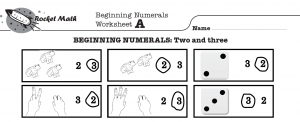Here is part of the Rocket Math–Beginning Numerals Learning Track: Worksheet A. This shows the top part of the worksheet which is the “I do” or teacher demonstration part of the worksheet. The teaching suggestion for the “I do” step for the teacher reads as follows:

Using the six boxes at the top of each worksheet, model how to touch
and count the items in each box–then show the students that the correct
answer is circled. Have them touch the circled number. Confirm the
name of the numeral. Check to see that all students are touching it.

At a small table for instruction, the teacher could hold the worksheet up on a clipboard, or for a larger group, a document camera would be needed. The teacher gets all eyes on the demonstration and then models touching and counting. Skilled kindergarten teachers know to be quick, perky, and interesting to keep their students attention. Here’s the recommended narration:

I can do this. Watch me count. One, two, three.
There are three frogs in this box. So they circled the three.
Touch here where the three is circled next to the three frogs. Good.
How many were in this box, everybody? Yes, three.
Now watch me do the next box.

Watch me count. One, two,.
There are two frogs in this box. So they circled the two.
Touch here where the two is circled next to the two frogs. Good.
How many were in this box, everybody? Yes, two.
Now watch me do the next box.

### Step 2–the “We Do” step: Lead the students to make the correct responses

Once kindergarteners can say the numbers by rote, the hard part is to develop the one-to-one correspondence between the words and the touching. This is much more difficult to do correctly than you might think. For starters, counting to ten by rote may seem like to little ones like a couple of long words, “Onetwothreefourfive sixseveneightnineten.” To teach them to touch each object and say only one word for each, the task must be done together, slowly and correctly, many times.

##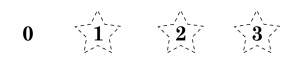#### We Do: Narration to Count Objects in an Image

Every worksheet in the Rocket Math Beginning Numerals Learning Track has a “We Do” activity of counting stars.  Here’s the recommended narration for the counting star’s activity pictured above entitled “We Do:”

Our ‘We Do’ says to touch and count. Start at zero and count each star.
We are going to touch and count the stars. Put your counting finger on zero,
everybody. We are going to start at zero and count each star. Let’s count.
One, two, three. We counted three stars. That was great!
Let’s do it again! Fingers on zero, everybody. Let’s count. One…

It doesn’t take much imagination to see that students will get this counting exercise right with the teacher’s help. If not on the first pass, by the third time, students will be correctly counting the stars. This counting exercise occurs on every worksheet in this Learning Track and gradually builds to counting up to 12 stars.

##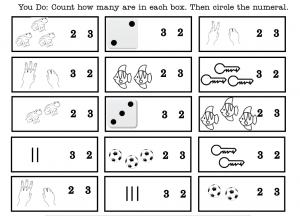In the “You Do” portion of Worksheet A of the Rocket Math Beginning Numerals Learning Track (see above) it asks students to count the objects and select, rather than produce or write, the correct number for the number of objects they see in the box. This is identical to the teacher demonstration exercise at the top of the worksheet. The teacher has demonstrated how to do this task already. Students just need to remember that from the demonstration a few minutes prior.

Students are asked to make that distinction, to choose between two and three, by circling the right one–as was done in the top six boxes in the teacher demonstration part of the worksheet. The demonstration answers at the top of the worksheet will be available for students to use as a model of the correct answer. Students learn from practicing the correct answer rather than from practicing errors, so error-free work is the goal.

## Benefits of using counting objects worksheets for kindergarten

### Counting objects worksheets can focus on one thing to learn at a time

In the “I do” worksheet demonstration, the teacher is helping students develop one discrimination and one only.  The task is learning to discriminate between counting two objects vs. counting three objects. The three different pictures demonstrate this; frogs, dice, and fingers. It becomes clear that the job is to get it right, to be able to tell the difference between two and three–that’s all. Students can learn one distinction like this with a high level of success.  The worksheet allows the teacher to move back and forth between two objects and three objects quickly.  Imagine how chaotic that would be to accomplish with manipulatives!

### Counting objects worksheets can transition students to fingers and lines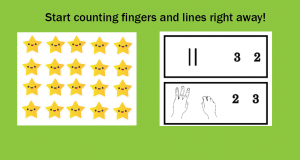Many lists of objectives include counting objects to 20. While the ability to count things up to 20 is important, that’s a lot of pictures. It is very easy to make a mistake in counting. It is much preferable to transition students to counting fingers, up to 10, and then to lines for numbers up to 20. A good worksheet program should include from the beginning pictures of fingers and lines to count instead of just cute objects. By introducing fingers and lines from the beginning, students will find it easy to transition to using only fingers or lines later.

### Worksheets can properly space out the objects to be counted

A common issue in counting objects is to have the objects spaced far enough apart so that students are reliably not touching the same object twice in a row. In the “We do” portion of the worksheet, pictured above, it is clear that students will succeed by beginning with three stars spaced far enough apart that students have to move their fingers to count the next star. They won’t be confused as they can be by counting objects that are too close together.

### A worksheet can establish a starting point for fingers before counting begins

Another problem in teaching counting is that students often begin counting by touching one of the objects they are counting. Then to begin counting they move to the second object and count, “One.” The end result will be off by one. Students must be taught to start with their counting finger somewhere else, and then move to the first object to be counted while saying, “One.” The Rocket Math Beginning Numerals (counting objects) worksheets always have students start with their finger on “zero,” which is pretty useful as a starting place. That’s where you start before you begin counting.  I expect the savvy teacher will be saying, as students are getting their fingers ready to count, “We are touching the zero.  Have we counted any stars yet? No!  So we have counted ZERO stars.  That’s why we are touching the zero.”

### A worksheet can allow students to choose the correct answer rather than produce itCounting worksheets that begin by requiring students to produce, or write, the numeral of the answer are asking too much of beginning students. When the written answer is wrong the teacher has no way of diagnosing whether the student counted incorrectly or forgot how to write the numeral. Much better to begin by having students select the correct answer from a limited number of choices. Teaching students (1) how to write numerals and then (2) write them from dictation are two different skills that should be taught in different instruction.

### Worksheets can limit displays to numbers the students have learned.

Another advantage of worksheets is the ability to limit the examples to numbers of objects that students have already learned. When a teacher allows students to use manipulatives the ability to control the number of objects to count is lost. Instead, as shown in the “You Do” portion of Worksheet A, shown above, students only need to be able to distinguish two objects from three objects.

By having the answers present to choose from, the worksheet limits the distinctions that the students need to make. Many beginning kindergarteners confuse 4 and 5 early on. Therefore that distinction, between 4 and 5, should not be required until 1, 2, 3, and 4 are well established and at mastery. You see here on Worksheet A students are only required to distinguish between 2 and 3 objects. They make the same distinction between several examples of different objects.

## Rocket Math Beginning Numerals (counting objects) worksheets will start kindergarten students out successfullyThe Beginning Numerals Worksheets is the first of over 25 math worksheet Learning Tracks available as part of the Rocket Math Worksheet Universal Level Subscription. See all the other Learning Tracks available in the Worksheet Program. Go here to get a 60-day trial subscription for \$15 with a money-back satisfaction guaranteed offer direct from the author, Dr. Don

Every year, students enter a new math level with new math facts, but what should the students know by the end of each grade? How do teachers measure the success of their students? By using math benchmarks, teachers have a reference point to assess their students’ progress. Rocket Math Online Game and Worksheet Program are great tools to help students meet math benchmarks in every grade.

## What are Math Benchmarks?

Math benchmarks are standard reference points that can be measured and assessed. Teachers use benchmarks in math to help understand where their students are in their math education and know where they need to be in order to succeed in their grade level.

In 1st grade, students focus on learning addition and subtraction up to 20, whole number relationships and grouping, linear measurement and lengths, and geometric shapes.

#### 1. Addition and subtraction up to 20.

1st grade students learn strategies to build math fact fluency for adding and subtracting whole numbers up to 20.

#### 2. Whole number relationships and grouping in tens and ones.

1st grade students learn to compare the whole numbers at least to 100 and be able to understand and solve problems involving their relative sizes.

#### 3. Linear measurement and measuring lengths.

1st grade students learn to understand the meaning and process of measuring including the concepts of iteration and transitivity principle for indirect measurement.

#### 4. Compose and decompose geometric shapes.

1st grade students learn how to compose and decompose geometric shapes, like creating a quadrilateral by putting two triangles together.

In 2nd grade math students focus on developing students’ knowledge of base-ten notation, their fluency with addition and subtraction, standard units of measure, and analyzing shapes.

#### 1. Base-ten system

2nd grade students learn to count by fives, tens and multiples of hundreds, and learn to recognize which digits represent amounts of thousands, hundreds, tens or ones. E.g. 456 is 4 hundreds + 5 tens + 6 ones.

#### 2. Addition and Subtraction up to 1000

2nd grade students develop their math fact fluency in addition and subtraction up to 1000.

#### 3. Standard units of measure

2nd grade students learn standard units of measure and how to use rulers and other measuring tools.

#### 4. Analyze Shapes

2nd grade students learn how to examine shapes by their sides and angles, and decompose and combine them.

In 3rd grade students focus on developing their multiplication and division math facts up to 100, understanding fractions, understanding the structure of rectangular arrays and are, and how to analyze two-dimensional shapes.

#### 1. Multiplication and division math facts up to 100

3rd grade students develop their math fact fluency of multiplication and division of whole numbers up to 100.

#### 2. Fractions and unit fractions

3rd grade students learn unit fractions and fractions and are able to use them to represent numbers equal to, less than, or greater than one.

#### 3. Attribute of two-dimensional regions

3rd grade students learn to find the area of a shape which shows the students how to connect the area to multiplication and how to use multiplication to determine an area of a rectangle.

#### 4. Analyze and compare two-dimensional shapes

3rd grade students compare and classify two-dimensional shapes by their angles and shapes.

In 4th grade, students focus on developing their math fact fluency in multi-digit multiplication and division, learn math operations with fractions, and understanding geometric figures.

#### 1. Multi-digit multiplication & division

4th grade students understand place value to 1,000,000 and know how to apply multi-digit multiplication and multi-digit division to mentally calculate quotients.

#### 2. Fraction equivalence and operations

4thd grade students learn that two fractions can be the same (e.g. 12/16 = 3/4) and learn how to add, subtract, multiply and divide fractions.

#### 3. Geometric figures

4th grade students learn to describe, analyze, compare and classify two-dimensional shapes.

In 5th grade, students focus on developing fraction operations, learn operations with decimals to 100, and develop an understanding of volume.

#### 1. Math fact fluency of fraction operations

5th grade students learn to apply their understanding of fractions and fraction models to calculate sums and differences of fractions in addition, subtraction, multiplication, and division.

#### 2. Relationship between decimals and fractions

5th grade students learn to multiply and divide between decimals and fractions, and to compute quotients of decimals to 100.

#### 3. Volume

5th grade students learn that volume is an attribute of three-dimensional space and can be measured by finding the total number of units that are needed to fill the space.

## Not meeting Benchmarks: what should it mean?

When students score as “not meeting benchmark,” it means the school should provide some intervention to help the students learn.  When it comes to learning math facts, most students have had no opportunity to learn them in a systematic way. Unless they have had an extraordinary school or an unusual teacher, they have not received structured, systematic learning opportunities to memorize math facts. After three decades in schools, I know this to be the case. Without systematic practice and effort, most students will not meet math fact benchmarks. They won’t meet the Common Core expectation that students will “know [math facts] by memory.” Whose fault is that? It is not the student’s fault nor the parent’s fault.

Are you certain your fluency intervention is effective?

The teacher and the school should provide, not just any “intervention” but should provide an effective intervention. Some so-called “interventions” do not reliably produce increased fluency. An essential part of using an intervention is to measure its effectiveness. [That’s why IEP goals are supposed to have measurable short-term objectives!] The best way to measure the effectiveness of a fluency intervention is with timed, curriculum-based fluency assessments.

By measuring the same way for the same amount of time period, you can see if fluency increases. If the student can complete more items during the timed test each time you measure, you can see their fluency increase. When a vast majority of your students, or all of your students, improve in fluency, then you can be certain that the intervention is effective.

## Rocket Math Online Game is effective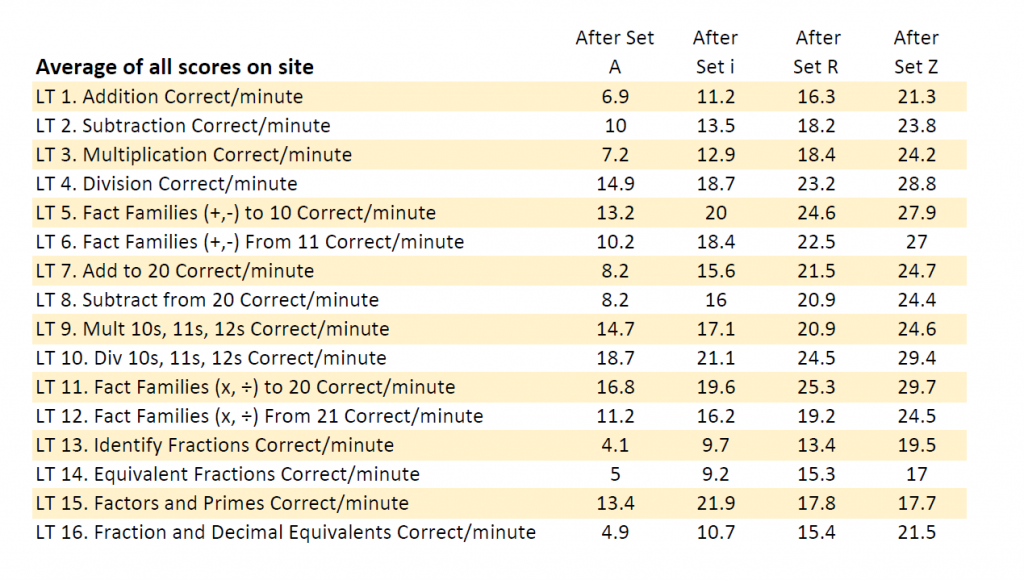As students work through the levels in each Learning Track in the Rocket Math Online Game, they are periodically assigned fluency tests.  Students are tested after completing levels A, i, and R, and then after they finish level Z. Each test is a 1-minute fluency test of a random selection of facts taught in that Learning Track. Therefore the scores are comparable, and when the number goes up at each point in the curriculum, you can be sure that students are increasing in fluency. As you can see from the site-wide data shown here, students consistently improve in fluency as they work through the Rocket Math Online Game. You would not expect students to meet benchmarks until they have completed Set Z in the Learning Track.

## Math fact fluency benchmark in the Online Game: 20/minute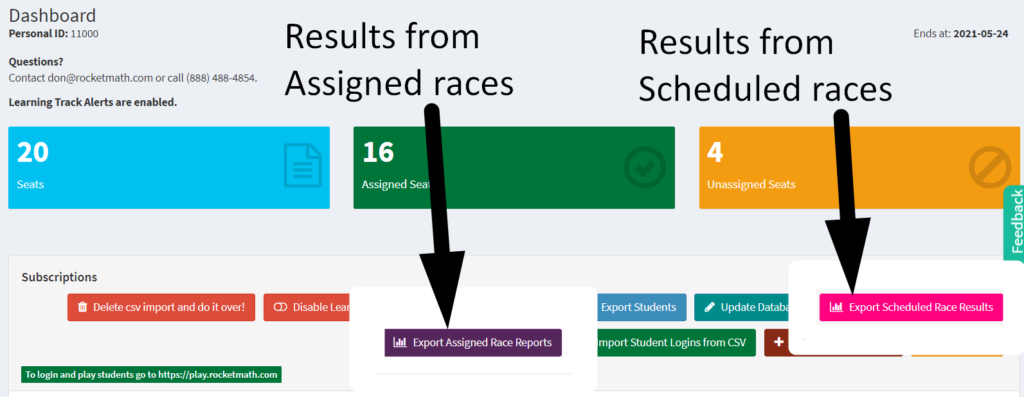The 1-minute RACEs in the Rocket Math Online Game are a good way to measure math fact fluency. You can see from the site-wide averages above that on average, students exceed 20 per minute correct at Set Z, and the average at the beginning is much less. So a reasonable benchmark is 20 correct problems per minute.

The teacher can assign a 1-minute RACE at any time, and the student will need to do it upon their next login. The results will be available in the Review Progress screen and export from the button that gives “Results from Assigned races.” However, the best measure of whether students can meet the benchmark is after Set Z, when they have completed the Learning Track. By clicking on the pink button for exporting “Results from Scheduled races,” the score will appear.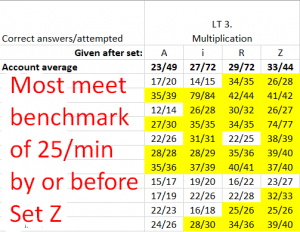To the right is a screenshot of an example of the results from scheduled races from one class of upper elementary students. You can see the Account Average shows improvement at each level. If you set a benchmark at 25, you see all but one of the students met that by the end of Level Z. However, we do see several students who began above that level. (They need not have gone through the Learning Track, but most improved anyway.) After Set Z, those scores show that nearly all students are proficient by the end of the Learning Track. So we know that the Online Game is an effective intervention. But there’s a catch. For this display, we eliminated a lot of students who had not completed Set Z in Multiplication. Students have to play the game to learn.

## Students must participate to learn: Monitor and Recognize Effort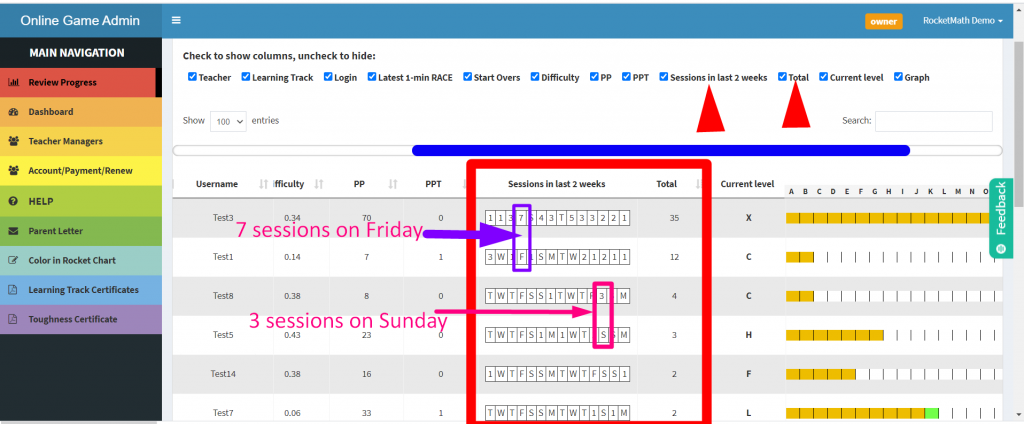Assigning an effective intervention will not help unless students are engaged and participate. Instead of reporting on benchmarks of academic achievement, why not report on benchmarks of effort? Each time students login and complete a session (five, ten, or fifteen minutes in length) on the Online Game, the Review Progress screen will record their session. A mini calendar shows the number of sessions per day. As shown in this screenshot, one student completed 7 sessions on the previous Friday. Another student completed 3 sessions on Sunday. The students below the hard workers, whose mini-calendars showed “F” for Friday and “S” for Sunday, did not complete any sessions because there was no number for those days.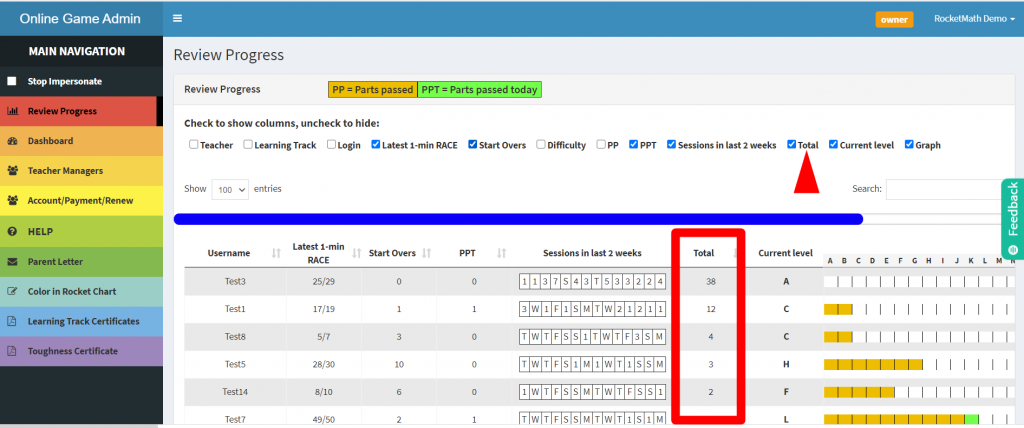Even more discriminative, the Review Progress screen shows the total number of sessions completed in the last two weeks. The picture shows data from our demo accounts. The one for Test3 shows 38 sessions in the last 14 days, while others have only 2 sessions. This total data tells us the level of effort students have been putting forth to learn their math facts. If you monitor this number and recognize students putting forth great effort, you’ll get more students participating. I would say that 10 to 14 sessions in the last two weeks are a good effort. 15 to 24 sessions is a very good effort. Any student with 25 or more sessions in the last two weeks is putting forth great effort and should be recognized as a star!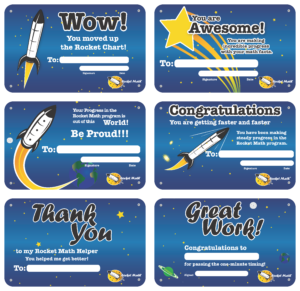You might consider giving out Award Certificates once a month for students who are putting forth super-star effort. If you reward effort, we guarantee you’ll get achievement. We have sets of Award certificates available on our supplements store site. Soon after doing that, you’ll probably have to start awarding Learning Track certificates for students who are completing Learning Tracks. Those are available on the Admin page on the light blue tab in the main rainbow navigation bar.

## Using Rocket Math to Help Students Reach Math Benchmarks

Rocket Math Online Game and Worksheet program will help students reach each grade level math benchmark. By systematically teaching students to be math fact fluency, they will be able to not only succeed but learn to enjoy math.

By 4th grade, students need to stop counting on their fingers and be able to recall math facts immediately. If not, then they will find it hard to learn multiplication and division. Rocket Math offers 4th grade math worksheets that will push your students towards success and prepare them for 5th grade.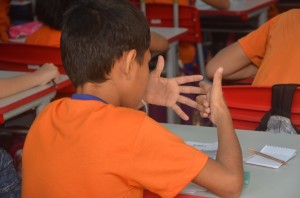## What should a 4th grader know in math?

By the end of fourth grade, students should have memorized all four basic operations of math facts: addition, subtraction, multiplication, and division. Typically, students in fourth grade learn division. However, if your fourth grade students haven’t mastered multiplication, you must help them develop multiplication math fact automaticity first and then teach them, division, if there is enough time in the school year. Without math fact fluency in multiplication, students will struggle with division and learning all higher-order forms of math including algebra.

The only way for students to successfully memorize math facts is systematical. Practicing random math facts or playing math games will never help students memorize. They will simply count the facts out over and over again–coming to hate math.

## How how to measure math fact fluency

Not sure if your students are fluent in multiplication math facts and are ready to learn division? If you see a student counting on their fingers, it’s a sure bet they have NOT memorized these basic single-digit facts. If you don’t know for sure, here’s our free resource with all you need to pre-test students on math facts.

## Why Rocket Math Worksheets help students develop math fact mastery

Rocket Math’s Worksheet Program is very different from any other math facts practice worksheets.

The program only teaches a couple of math facts at a time, helping students succeed every day.

Once students learn the correct answers, the Worksheet Program includes a lot of fast-paced practice. With this “learn first, practice second” method, students easily commit their math facts to memory.

The program is graduated and progressive. It mixes newly memorized math facts with previously memorized math facts, helping students develop over time the ability to answer more than 400 math facts instantly from recall.

When students develop automaticity (facts so well learned that their recall is instantaneous), all math becomes easier.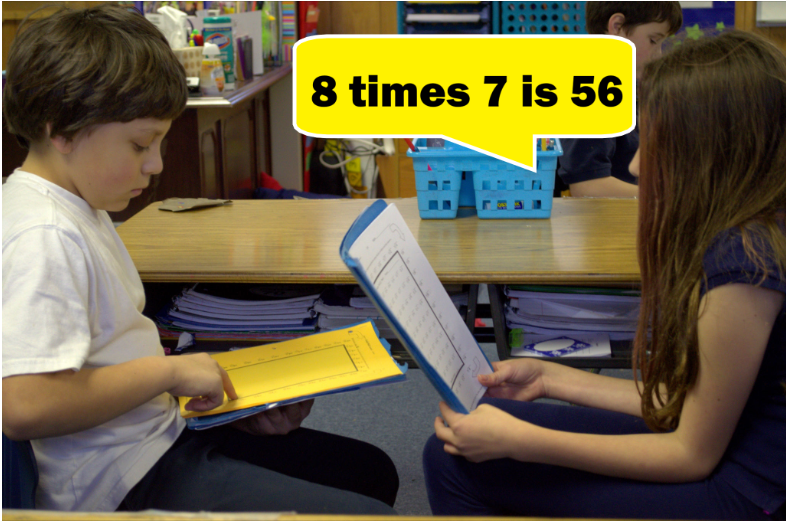A unique component of the Worksheet Program is oral student practice. This allows students to receive corrective feedback from their partner, who has the answer key. You cannot learn without corrective feedback from either a person or a computer. Having teachers give corrective feedback by checking and grading written practice is very time-consuming, as well as being too late for effective learning. Having student partners with answer keys correct immediately, during practice, means teachers only have to grade check-point tests instead of monitoring daily practice–a huge plus for time-strapped teachers!

The Worksheet Program is designed to build strong multiplication fact fluency and recall. Memorizing these facts avoids the problem of students having to look up facts in times tables, over and over again.

## How to teach MULTIPLICATION with Rocket Math’s 4th Grade Math Worksheets

Since many students begin fourth grade without mastery of multiplication facts, let’s start with Rocket Math’s first multiplication worksheet of Set A. As you can see, the worksheet has only “1 times” problems, such as 3 times 1, and 1 times 2. These are very easy, and students can typically answer them quickly, with no hesitation.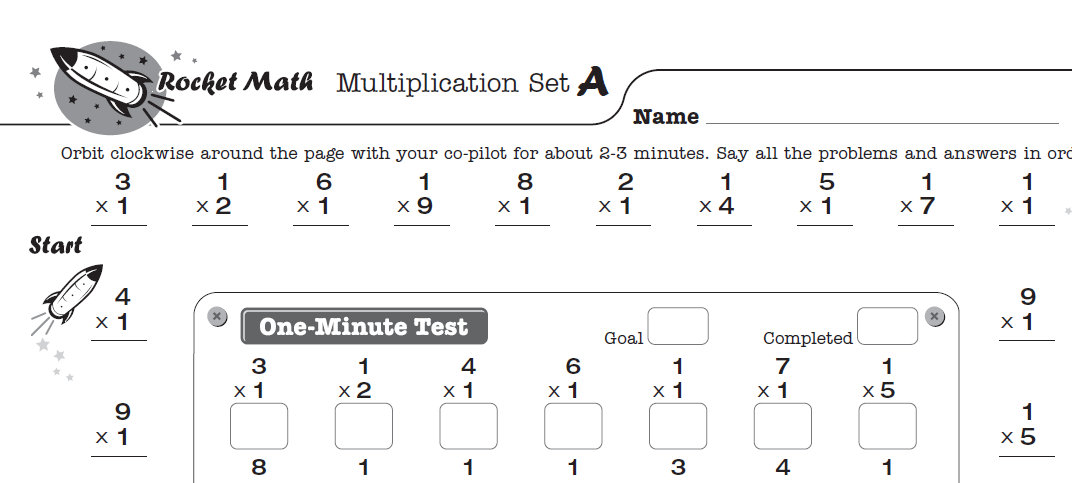First, students practice orally with a partner around the outside of the worksheet. For example, one student practices saying the problems and the answers while the other student offers corrections. You need to teach students that the checker’s role is to correct any fact that isn’t answered instantly without hesitation. The checker needs to follow this special correction procedure.

After two to three minutes of oral practice, students should switch roles and help their partner practice.

Finally, all students take a one-minute test of these facts on the inside of the worksheet. They will be able to write the answers to those facts as fast as their fingers can carry them.

## Writing Speed Test

Before beginning the Worksheet Program, students need to establish goals with a writing speed test. Students who can answer the problems in the one-minute daily test at their established speed will pass on to learn the next set of facts the following day.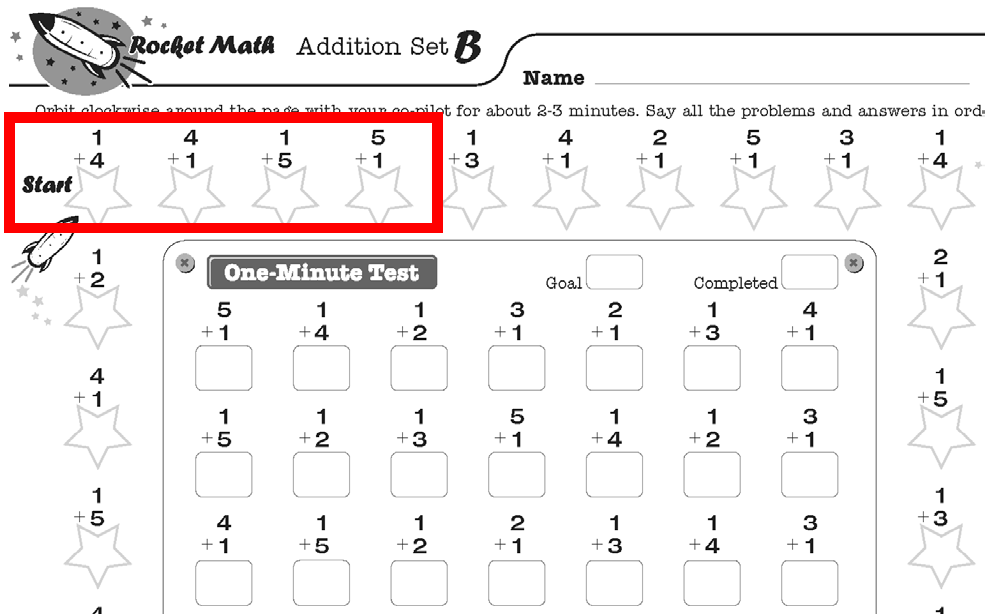Once your students master Set A, move on to Set B, then Set C, and so on. Get all of the Multiplication 1s to 9s worksheets from rocketmath.com. Each worksheet follows the same procedure:

1. Students practice saying the answers to the “outer ring” problems out loud with a partner.
2. Partners follow the special correction procedure for any fact that isn’t answered instantly without hesitation.
3. After 2-3 minutes of partner practice, all students take a one-minute test of the recently learned facts located on the inside part of the worksheet.
4. Students that answer the correct amount of math fact problems in 1 minute (based on their writing speed) progress to the next worksheet set. Students that fail the test, repeat steps 1-4 using the same worksheet set the following day.

It usually only takes students a few days to memorize a set.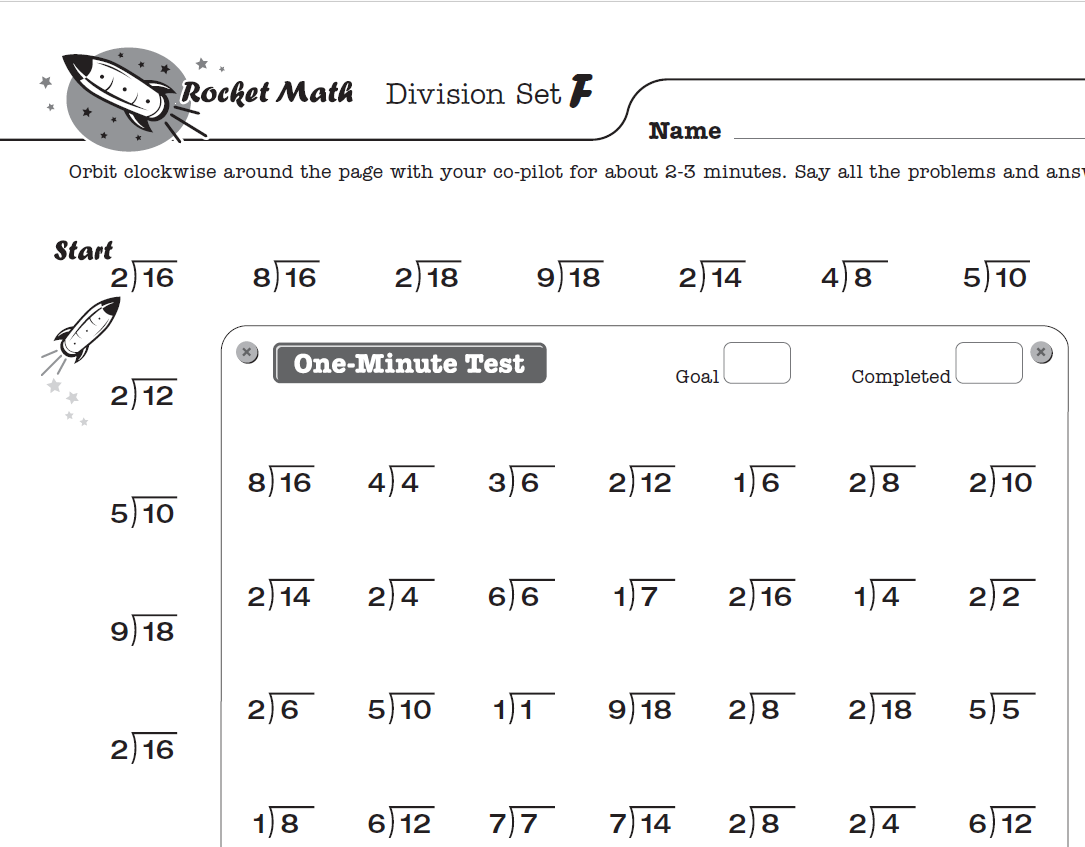## How to teach DIVISION with Rocket Math’s 4th Grade Math Worksheets

Once your fourth graders master multiplication math facts, it’s time to teach them division.

To teach your fourth graders division with Rocket Math’s worksheets, use the Division 1s through 9s worksheet and follow the same procedure described in the previous section on multiplication.

The Common Core suggested that students learn math facts up through the 12s. Rocket Math has two Worksheet Programs that do just that. One is Multiplication 10s, 11s, 12s. and the other is Division 10s, 11s, 12s. Each builds upon and reviews facts from the 1s-9s program while keeping learning fun for students who learn faster and need additional challenges.## Fact Family Worksheets are another way to study facts

Fact families worksheets provide an alternative way for fourth graders to learn multiplication and division facts. A fact family in multiplication and division is 4 x 3, 3 x 4, 12 ÷ 3, and 12 ÷ 4. By learning these four facts simultaneously, students can internalize the reciprocal nature of multiplication and division. This is also a viable way for students to learn facts initially if that is the teacher’s preference. This can also be a great review for students who begin the year having mastered multiplication and division.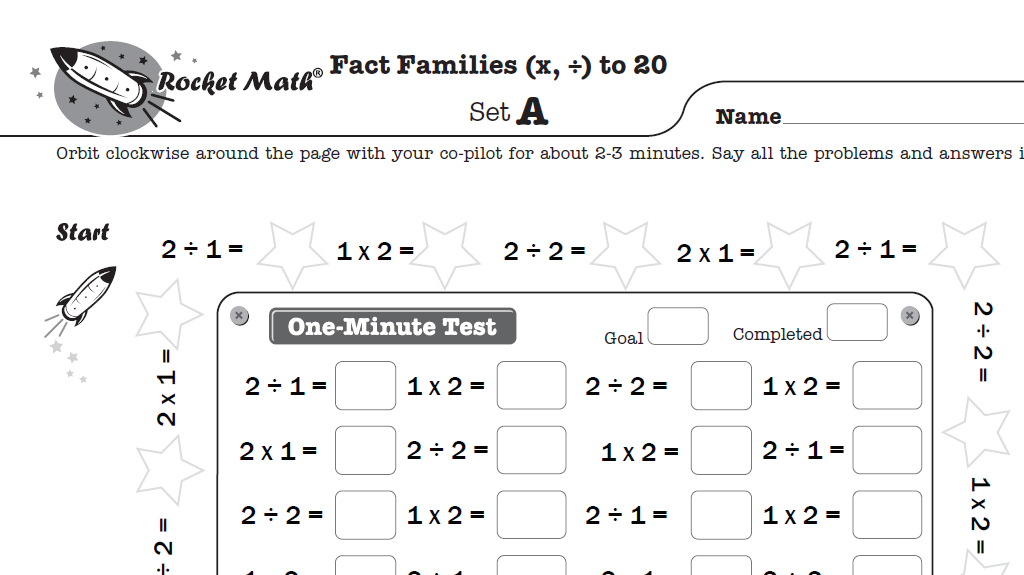### Rocket Math Worksheet Program’s specially designed Fact Families

Much like Rocket Math’s single operation worksheets, each Fact Family worksheet in the Rocket Math program asks students to memorize only one fact family at a time. You can see the first fact family students learn in Set A is 1 x 2, 2 x 1, 2 ÷ 1, and 2 ÷ 2. Each set in this program introduces only one fact family to help students easily memorize fact families and work quickly through Sets A to Z..

Rocket Math’s Worksheet Program starts with the first level of Multiplication and Division Fact Families to 20, followed by Multiplication and Division Fact Families from 21. Practicing with fact families reinforces the reciprocal nature of multiplication and division and gives extra practice to increase fluency.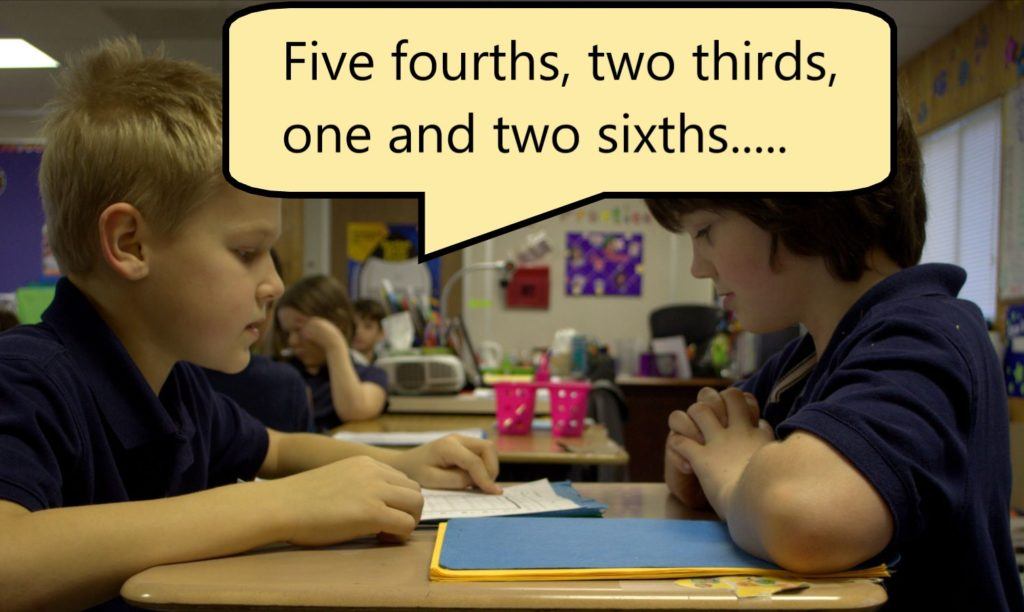## 4th grade worksheets for learning fractions and factor pairs

Rocket Math Worksheet Program works through a daily routine of oral practice with peers and one-minute tests to determine how fast a student should progress through the A to Z steps. By establishing a daily routine, students can use it to study fractions, factors, and decimals in the more advanced pre-algebra programs available in Rocket Math. These will seriously give students a “leg-up” when they come to pre-algebra concepts that students find slow or cumbersome.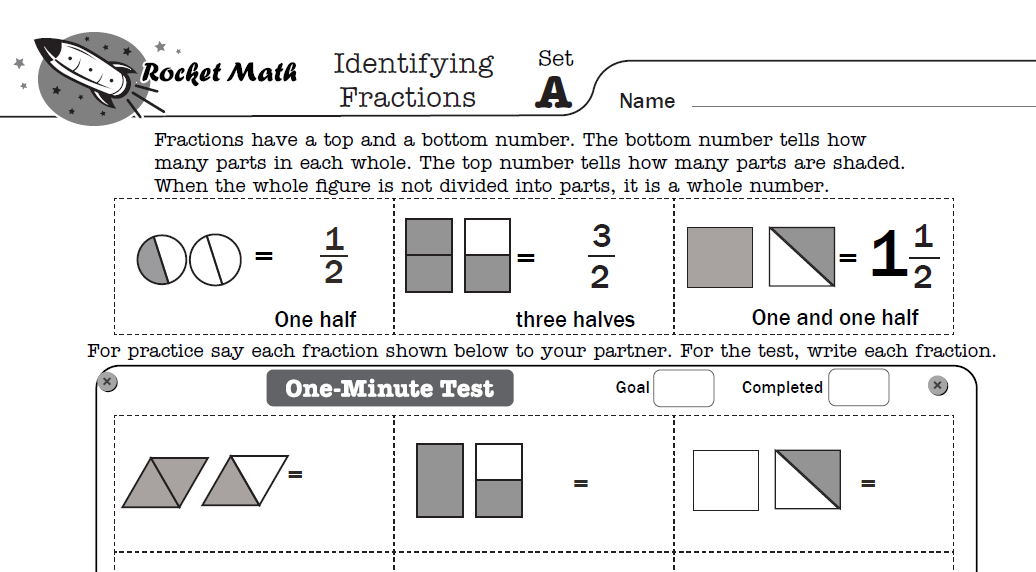### Identifying Fractions Worksheets

One such Rocket Math program is the Identifying Fractions Worksheet program. In it, students learn to identify pictures of proper fractions, improper fractions, and mixed numbers. Each A to Z set teaches a new set of three closely related fractions so that students learn to identify the fractions quickly and easily. Students learn this rule: Fractions have a top and a bottom number. The bottom number tells how many parts in each whole. The top number tells how many parts are shaded. When the whole figure is not divided into parts, it is a whole number. The program also presents whole numbers to identify mixed in with fractions. If you have ever seen students struggle with identifying fractions when they are looking at them, you’ll appreciate the power of these worksheets.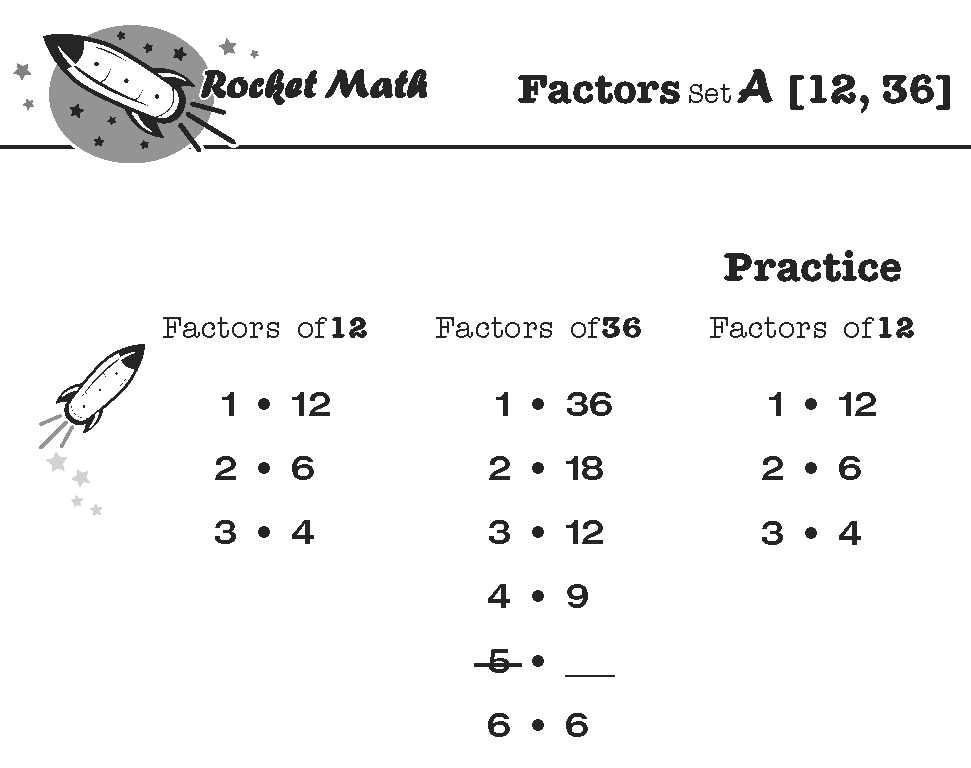### Factors (how to find all the factor pairs) Worksheets

Rocket Math Worksheet Program Factors have worksheets to quickly teach students how to name all the factor pairs of common numbers. What are the factors of 36? Answer: 1 x 36, 2 x 18, 3 x 12, 4 x 9, 6 x 6. This is what students learn by memory. Here’s a blog about the foolproof method that students learn. Students practice with a partner, take a daily one-minute timing, fill in a Rocket Chart, just like regular Rocket Math. Simplifying fractions will be a breeze after students learn this easy method and practice finding all the factors of these common numbers.

Students learn all the factors for these numbers in this sequence: 12, 36, 24, 48, 18, 32, 16, 64, 10, 40, 20, 72, 8, 25, 50, 6, 21, 30, 60, 15, 45, and 100. Click here to learn “How to Factor” from Dr. Don’s Educreations lesson on the web.

### Equivalent Fractions

Students need to know that six-eighths is equivalent to three-fourths and that four-twelfths is equivalent to one-third. While they can calculate these, it is very helpful to know the most common equivalent fractions by memory. One of the most common problems students have with fractions is not “reducing their answers to simplest form.”

Here’s a 5-minute Educreations lesson on How the Equivalent Fractions program works.

The Equivalent Fractions Worksheet Program is part of the Universal Level subscription to the Worksheet Program. Equivalent fractions will help students commit 100 common equivalent fractions to memory. Each set (A through Z) has four fractions, which are displayed on a fraction number line. Students frequently learn fractions equivalent to one, such as ten-tenths, and fractions that can’t be reduced. For example, three-fourths is equivalent to three-fourths. Using the fraction number line will help with students’ understanding of why those fractions are equivalent.

Click here for the full sequence of 100 Equivalent fractions that students will learn in this program.

## Start Rocket Math’s Worksheet Program today

Rocket Math’s unique worksheet program is not only great at teaching fourth graders multiplication and division, but the program also offers beginning numerals, addition, subtraction, and pre-algebra concepts for ages K-12. Each worksheet follows the same effective methodology described in this article and is guaranteed to help your students memorize math facts. Get a Rocket Math Worksheet Subscription for your school or home today.

## What Math Facts Should Students Know By 2nd Grade?

Students entering 2nd grade should have memorized basic addition facts to a high level of fluency. There should be no hesitations and no finger counting. But not every first-grade teacher has the skill or tools to help students effectively memorize addition facts.  Attempting to teach subtraction facts and the process of subtraction to students who did not successfully memorize addition is VERY difficult. Like, so hard as to cause tears, and not just from the students! Rocket Math offers 2nd grade math worksheets and 2nd grade math games.

## What is Covered in 2nd Grade Math?

In 2nd grade, teachers need to help students master at least four math skills in three steps. It’s important that 2nd graders learn these skills in the correct order because each step is dependent on the completion of the prior step.

### Step 1. Fully memorize addition facts.

Second-grade teachers must ensure that students have fully memorized the basic addition facts. Most 2nd grade math games will not accomplish this goal. Memorizing addition facts early in the year is crucial because the class needs time to do the next two steps during the year. For this reason, the 2nd-grade teacher needs a systematic, effective, and efficient math facts learning curriculum, such as Rocket Math. If the 2nd-grade teacher is lucky, that was accomplished during first grade. If it was not, it still must be done.

### Step 2. Master addition computation while memorizing subtraction facts.

Second-grade teachers must ensure that students master addition computation up to three digits. If students are automatic with the addition facts, this process becomes easy and fun for students. Rocket Math’s Learning Addition Computation worksheet program is a systematic way of teaching computation skills.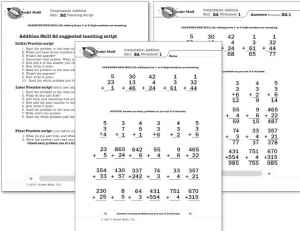The 2nd grade teacher also needs to help their students memorize basic subtraction facts. Learning subtraction facts should not be attempted until students have memorized addition facts fully. Because time is of the essence, a teaching tool with a systematic method of teaching facts efficiently and effectively is needed. Rote memorization is the best method. However, the teacher can have students work on memorizing subtraction facts while working on addition computation.

### Step 3. Master subtraction computation.

Second-grade teachers must ensure that students master subtraction computation up to three digits, with and without regrouping. If students are automatic with the subtraction facts, this process becomes easy and fun. Rocket Math’s Learning Subtraction Computation worksheet program is a systematic way of teaching computation skills.

###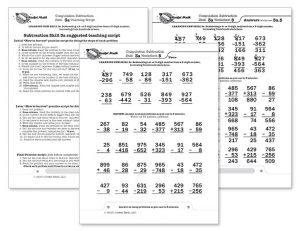Before going into 3rd-grade, students should be able to read and write numbers up to 1,000 and have developed a comfortable number sense of numbers up to 100. 2nd graders need to memorize addition and subtraction facts and be comfortable completing addition and subtraction computations. Before 3rd grade, 2nd graders need to start learning how to skip count as a precursor to learning multiplication and have learned the concept of multiplication (be able to figure out a multiplication fact). Rocket Math has a worksheet-based version of Skip Counting that students enjoy. They also sell Skip Counting Flashcards, which I’ve never seen anywhere else.

## Rocket Math 2nd Grade Math Worksheets & 2nd Grade Math Game

Both Rocket Math Worksheet Program and Rocket Math Online Game are great tools that will help students learn their math facts. Each program has a different way of teaching, but the idea and ultimate goal for both are the same; students learn math fact fluency and can recall answers instantly. Teachers can choose one or both of these programs to help their 2nd grade students be successful in math.

### H3: 2nd Grade Math WorksheetsThe Rocket Math Worksheet Program is uniquely effective by using paired practice and having students saying the facts aloud. Students partner up and practice quickly recalling facts together. One student reads the problems and answers from memory. The checker watches for when their partner hesitates to answer. He or she then gives his or her partner more opportunities to practice the “hesitant” facts.

The students switch roles, and after both have answered questions, they take a one minute test on the facts that they have learned so far. If students answer as fast as their fingers will carry them, they pass the level and move on to the next worksheet in the sequence.

#### Learn Addition and Subtraction facts with  these Worksheet Programs for 2nd Graders

For 2nd Grade math students, it’s essential that they master addition math facts and begin learning subtraction math facts. Here are the addition and subtraction fact Worksheet Programs 2nd graders will need to work through. They are listed in the order of priority.  Few students will finish all six in one year, but the more they accomplish the better they will know these facts.

 (1) Addition (1s to 9s) (3) Fact Families (+, -) to 10 (5) Add to 20  (e.g., 13+6, 4+11, 15+5) (2) Subtraction (1s to 9s) (4) Fact Families (+, -) from 11 (6) Subtract from 20 (e.g., 18-15, 15-5, 19-8)

### Rocket Math Online Game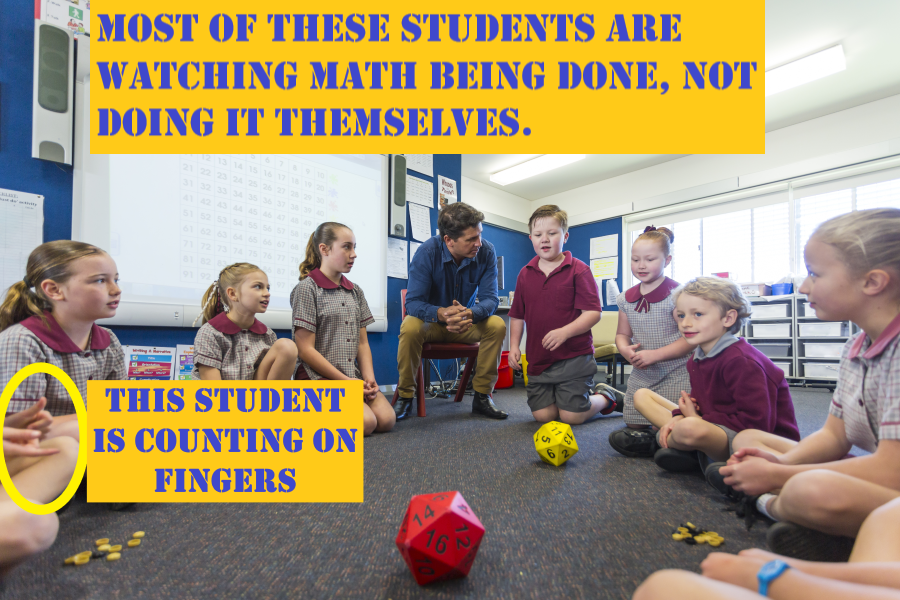In addition to worksheets, schools of education tell teachers to use games to “teach” math facts. Unfortunately, most games and fun activities do not help individual students learning math facts to the level of fluency. These games, such as bingo or dice, have several problems:

•  Students spend most of their time waiting for their turn rather than practicing facts.
• They do not focus on teaching a small group of facts in a manner that helps students commit them to memory.
•  The games do not adjust to an individual student’s level of fluency.
• Students can pace the game slowly enough to have time to figure out facts rather than requiring recall.
•  It is difficult to keep every student engaged, as those behind are less likely to participate.

#### Rocket Math Online Game is an Effective Way to Teach Math Fact Fluency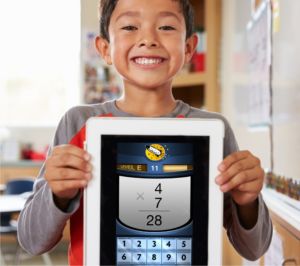Unlike card or dice games, some online math games are very effective at building math fact fluency. Games such as the Rocket Math Online Game have several important features that make a big difference.

1. Every student is engaged —not waiting for a turn.
2. Students learn only a few facts at a time, enabling memorization and recall.
3. The game provides lots of focused practice on each set of facts.
4. Students only get enough time to use recall to come up with the answers.
5. The game gives an immediate correction and extra practice on any facts that students cannot answer quickly.
6. The game only introduces new facts once students demonstrate mastery of prior facts.
7. The game gives students explicit feedback so they have a sense of accomplishment.

#### Learn Addition and Subtraction facts with  these Online Game Learning Tracks for 2nd Graders

Compared to the Worksheet Program, the Online Game moves students through the Learning Tracks much faster–in a few weeks, rather than months. Therefore you can send every one of the same age through in the same order. For 2nd graders, this is the online game learning track recommended by Rocket Math:

• Subtraction (1s – 9s)
• Fact Families (+, -) to 10.
• Fact Families (+, -) from 11.
• Subtract from 20.

2nd grade math games are a great way to teach students their addition and subtraction facts. Just be sure to find ones like Rocket Math that understand the importance of fact fluency and instant recall.

## A Data-Proven Approach to Measuring Math Facts Fluency (That Kids Love!)

You know your student is building math facts fluency, but can you quantify it? With Rocket Math’s Online Game, you can track your student’s progress learning addition, subtraction, multiplication, and division (and 12 other Learning Tracks) from level A to Z. Progress is tracked numerically through 1-Minute Races so that you can compare results over time, between students, and across classrooms.

## How Rocket Math’s 1-Minute Races Measure Math Fact Fluency

Starting in the Fall of 2020, the Rocket Math Online Game began scheduling 1-Minute Races for students as they worked through the 16 different Learning Tracks taught in the game.

The 1-Minute Races present a random selection of all the facts in each Learning Track. Students are encouraged to skip facts they haven’t learned, but the scores shown here are the correct answers. When a student completes sets A, i, R, and Z, the 1-Minute Races (also known as math facts fluency tests) are scheduled. We wait until after students complete set A to give the first pre-test, so we know the students know how to use the interface and answer questions.

As the chart below shows, Set A represents the average student’s starting score. Set i and R show intermediate progress. And finally, Set Z represents the average fluency students have achieved by playing through levels 26 A to Z in that Learning Track.

#### Average 1-Minute Race Scores of Students Playing the Rocket Math Online Game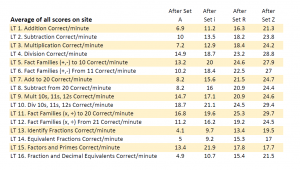The more correct problems per minute, the more fluent the learner. On average, by the end of a Learning Track students should aim for 20 to 30 correct problems per minute in the Online Game races. Want to learn more about how fast students should be with math facts? Read here.

## Data show improved math facts fluency as students work through each Learning Track

As you can see above, there is a steady rise in fluency as students work through each Learning Track. Because fluency scores go up consistently at each measuring point, and they do so in every Learning Track, we can be sure that the Online Game is effectively developing fluency.

## Data shows improvement based on how many math facts answered per minute

The fact that on each teststudents can correctly answer more problems shows that they are learning, and the data proves it. That is very important, as you don’t purchase this game simply to entertain children. The goal is to help students achieve math facts fluency, and we are seeing that here. Individual results will, of course, vary.

## Not only an excellent online math speed test but also an excellent tool for research

Teachers can also assign these 1-Minute Races at any time, or even on a weekly basis. The scheduled tests, however, show the best effect of the Online Game because the amount of work done to achieve the tests is equal among students. Both scheduled and teacher-assigned results are kept, and the results of these tests can be exported in excel files, so they are available for research purposes. We invite you to study your students’ math fact fluency using the Rocket Math Online Game and 1-Minute Races. One thing is certain from our data, the Rocket Math Online Game works!